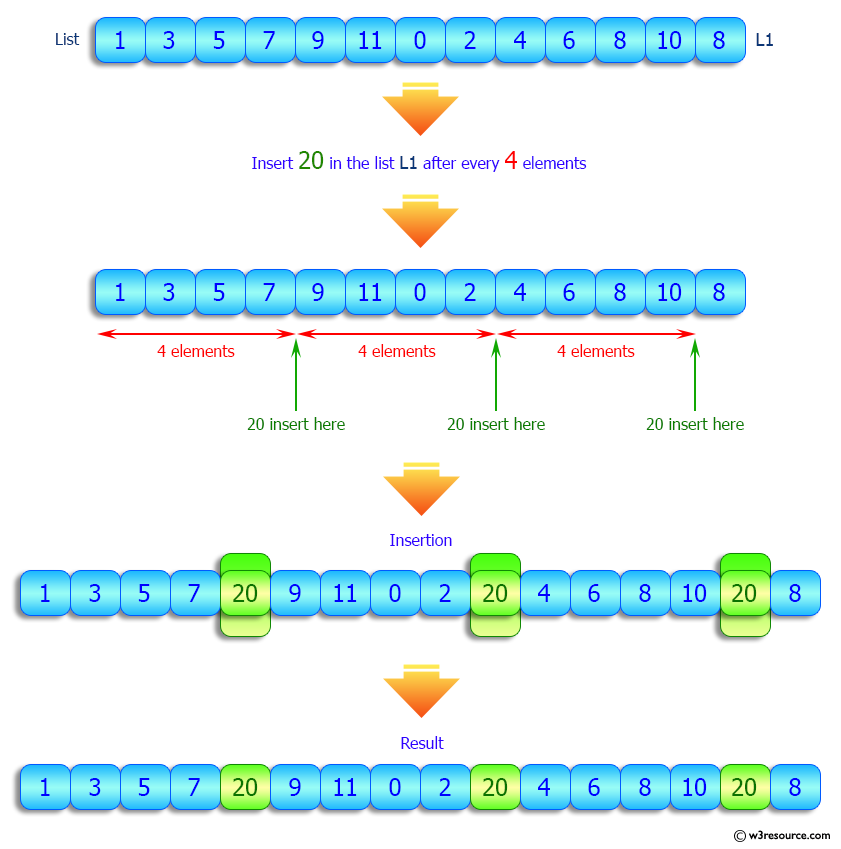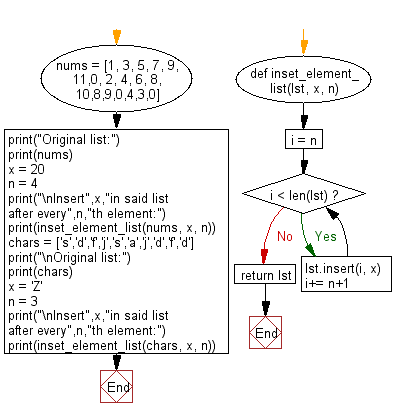﻿ Python: Insert a specified element in a given list after every nth element - w3resource# Python: Insert a specified element in a given list after every nth element

## Python List: Exercise - 178 with Solution

Write a Python program to insert a specified element in a given list after every nth element.

Sample Solution:

Python Code:

``````def inset_element_list(lst, x, n):
i = n
while i < len(lst):
lst.insert(i, x)
i+= n+1
return lst

nums = [1, 3, 5, 7, 9, 11,0, 2, 4, 6, 8, 10,8,9,0,4,3,0]
print("Original list:")
print(nums)
x = 20
n = 4
print("\nInsert",x,"in said list after every",n,"th element:")
print(inset_element_list(nums, x, n))

chars = ['s','d','f','j','s','a','j','d','f','d']
print("\nOriginal list:")
print(chars)
x = 'Z'
n = 3
print("\nInsert",x,"in said list after every",n,"th element:")
print(inset_element_list(chars, x, n))
```
```

Sample Output:

```Original list:
[1, 3, 5, 7, 9, 11, 0, 2, 4, 6, 8, 10, 8, 9, 0, 4, 3, 0]

Insert 20 in said list after every 4 th element:
[1, 3, 5, 7, 20, 9, 11, 0, 2, 20, 4, 6, 8, 10, 20, 8, 9, 0, 4, 20, 3, 0]

Original list:
['s', 'd', 'f', 'j', 's', 'a', 'j', 'd', 'f', 'd']

Insert Z in said list after every 3 th element:
['s', 'd', 'f', 'Z', 'j', 's', 'a', 'Z', 'j', 'd', 'f', 'Z', 'd']
```

Pictorial Presentation:Flowchart:## Visualize Python code execution:

The following tool visualize what the computer is doing step-by-step as it executes the said program:

Python Code Editor:

Have another way to solve this solution? Contribute your code (and comments) through Disqus.

What is the difficulty level of this exercise?

Test your Python skills with w3resource's quiz

﻿

## Python: Tips of the Day

Floor Division:

When we speak of division we normally mean (/) float division operator, this will give a precise result in float format with decimals.

For a rounded integer result there is (//) floor division operator in Python. Floor division will only give integer results that are round numbers.

```print(1000 // 300)
print(1000 / 300)```

Output:

```3
3.3333333333333335```